# Campus 101

6864 Registered Allowed team size: 1

This campaign is over.

hackathon
Online
starts on:
Dec 06, 2020, 06:24 PM
ends on:
Dec 06, 2020, 06:25 PM

## DS - Milestone 2

#### Tree

• A tree is a data structure similar to a linked list. The major difference is that in a tree, each node points to a number of nodes.
• It is a non-linear data structure.
• It represents the hierarchical nature of a structure in a graphical form.

Important terminologies

Let’s consider the following tree: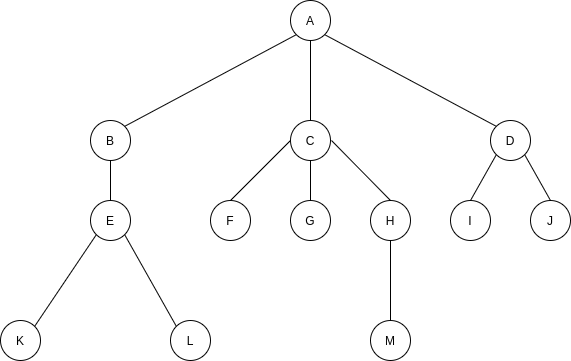• A is the root node and nodes B, C, and D are its children nodes. A can also be referred to as the parent of nodes B, C, and D.
• There can be at most one root node in a tree.
• An edge refers to the link from a parent node to its child.
• A node with no children is called a leaf node. Here, nodes F, G, I, J, K, L, and M are leaf nodes.
• Children of the same parent are called siblings. Here, nodes B, C, and D are siblings as are nodes F, G, and H.
• A node A is an ancestor of a node E if there exists a path from the root to E such that A appears on the path. The node E is called the descendant of A. For example, A, C, and H are ancestors of M.
• The set of all the nodes at a given depth is called the level of the tree.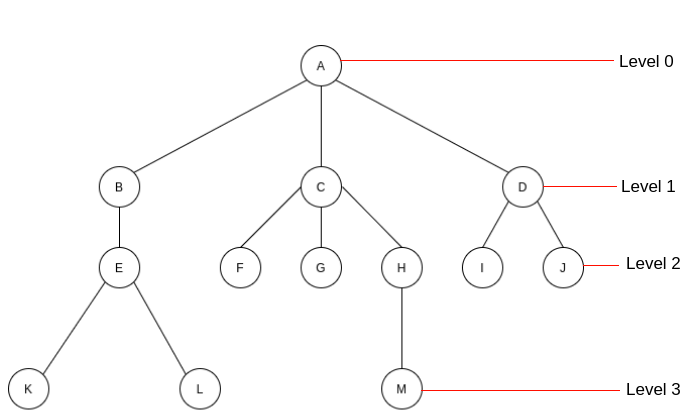• The depth of a node is the length of the path from the root to the node. For example, the depth of G is 2 (A-C-G).
• The height of a node is the length of the path from a particular node to the deepest node. For example, the height of C is 3 (C-H-M).
• The size of a node is the number of descendants it has including itself. For example, the size of C is 3.
• If every node in a tree has only one child (except the leaf nodes) then it is called a skew tree. If every node has only a left child then it is a left skew tree. Similarly, if every node has only a right child then it is a right skew tree.

You can refer to this to understand the concept of trees in detail.

Binary tree

• A tree is called a binary tree if each node has zero child, one child, or two children.
• An empty tree is also a valid binary tree.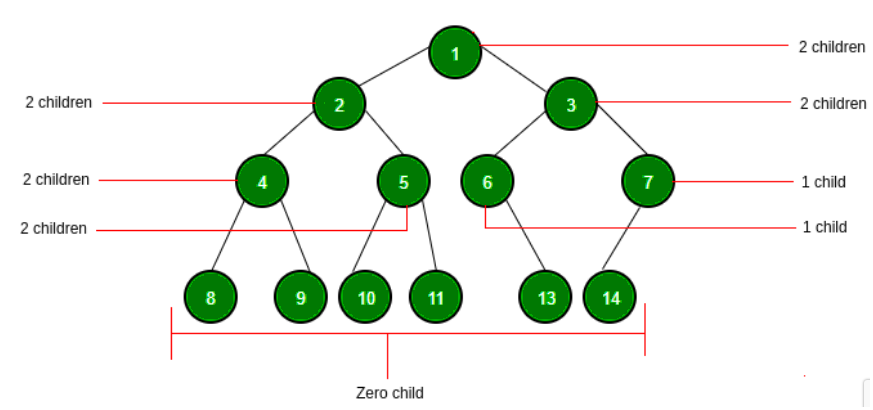Types of binary trees

• Strict binary tree: If each node has exactly two children or no children then such a binary tree represents a strict binary tree.
• Full binary tree: If each node has exactly two children and all the leaf nodes are at the same level then such a binary tree represents a full binary tree.
• Complete binary tree: Let the height of the binary tree be h. If all the leaf nodes are at a height h or h-1 without any missing numbers then such a binary tree represents a complete binary tree.

Read this to understand the types of binary trees in detail.

Binary tree properties

Let’s assume that the height of a tree is h.

• The number of nodes n in a full binary tree is 2^{h+1}-1.
• The number of nodes n in a complete binary tree is between 2^h (minimum) and 2^{h+1}-1 (maximum).
• The number of leaf nodes in a full binary tree is 2^{h}.
• The number of None links (wasted pointers) in a complete binary tree of n nodes is n+1.

Binary trees have some more properties that are used while programming. It is advisable to read this article that explains these properties. You can also solve problems for better understanding.

Structure of a Binary tree

One way to represent a node that contains data is to have two links. These links point to the left and the right children along with the data fields. The following code describes this scenario: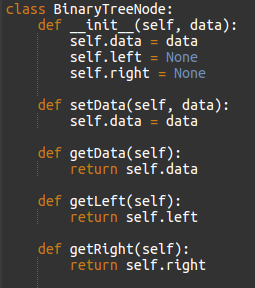Binary search tree

This is another variant of a binary tree. As the name implies, the main task of this tree is searching.

In a binary search tree, all the left subtree keys must be less than the root key. Also, all the right subtree keys must be greater than the root key. This is called the binary search tree property.

Note: This property must be satisfied at every node in the tree.

You can perform the following two types of operations on a BST:

• Main operations that include finding the maximum or minimum element, inserting an element, and deleting an element.
• Auxiliary operations that include finding K^{th} smallest element and sorting the elements.

There is no difference between the regular binary tree declaration and binary search tree declaration. (You can refer to the provided one in the binary tree section.)

Let’s try to find an element in a binary search tree. It is a simple and straightforward operation in BST. It is performed as follows:

You start with the root and move continuously towards left or right using the BST property.

• If the data you are searching is the same as the nodes data then return the current node.
• If the data you are searching is less than the nodes data then search the left subtree of the current node; otherwise, search the right subtree of the current node.
• If data is not present, return None.

The program is as follows: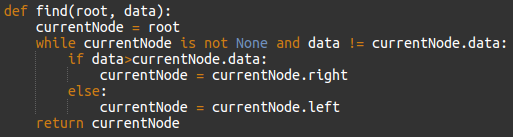You can refer to this to understand these operations in detail.

How will you find the maximum element in a binary search tree?

If you solve this problem, you can solve the practical problem easily!

Heap

A heap is a tree with the following property:

• The value of a node must be less than equal (or more than equal) to the values of its children. This is called the heap property.
• All leaves must be at h or h-1 levels for some h>0 (complete binary tree). Therefore, a heap must form a complete binary tree.

In the following diagram, the tree on the left is a heap (each element is greater than its children) whereas, the tree on the right is not a heap (since 2 is not greater than 4).

Types of heaps

Based on the property of heap, there are two types.

• Min heap: The value of a node must be less than or equal to the values of its children
• Max heap: The value of a node must be greater than or equal to the values of its children.

Binary heap representation

In a binary heap, each node can have at most two children.
Let’s assume that the elements are stored in arrays which start at index 1.

 Element 7 5 6 1 4 2 3 Index 1 2 3 4 5 6 7

Assuming that you are working with max heap, you can declare a heap in the following way: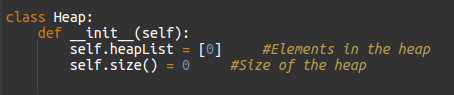Refer to this to learn about the various operations that you can perform on a binary heap.

Hashing

Hashing is a technique used for storing and retrieving information as quickly as possible. It is used to perform optimal search operations and in implementing symbol tables.

The hash table structure represents an unordered collection of associations between a key and a data value. The keys in a hash table are all unique. This allows a one-to-one relationship between a key and a value. You can perform the following operations:

• HashTable: Creates a new hash table
• Get: Searches the hash table with a key and returns the value if it finds the element with the provided key
• Delete: Deletes a key-value pair from the hash table
• DeleteHashTable: Deletes the hash table

Components of hashing

Hashing has the following four key components:

1. Hash tables
2. Hash functions
3. Collisions
4. Collision resolution techniques

For more details, refer to this.

Hashing vs array

In many applications you require arrays that are associated with each other. Such data are very complex and require a key to access them. For example, the key can be a student ID and the data entry can be a collection of grades. Simply using an array can make this scenario cumbersome. Hence, you can use hashing—the process of mapping the keys to available main memory locations.

You can read this concept in detail by reading Concept of hashing.